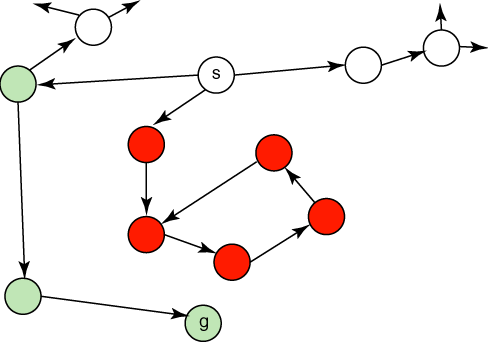## 3.6 Heuristic Search

All of the search methods in the preceding section are uninformed in that they did not take into account the goal. They do not use any information about where they are trying to get to unless they happen to stumble on a goal. One form of heuristic information about which nodes seem the most promising is a heuristic function h(n), which takes a node n and returns a non-negative real number that is an estimate of the path cost from node n to a goal node. The function h(n) is an underestimate if h(n) is less than or equal to the actual cost of a lowest-cost path from node n to a goal.

The heuristic function is a way to inform the search about the direction to a goal. It provides an informed way to guess which neighbor of a node will lead to a goal.

There is nothing magical about a heuristic function. It must use only information that can be readily obtained about a node. Typically a trade-off exists between the amount of work it takes to derive a heuristic value for a node and how accurately the heuristic value of a node measures the actual path cost from the node to a goal.

A standard way to derive a heuristic function is to solve a simpler problem and to use the actual cost in the simplified problem as the heuristic function of the original problem.

Example 3.12: For the graph of Figure 3.2, the straight-line distance in the world between the node and the goal position can be used as the heuristic function. The examples that follow assume the following heuristic function:
 h(mail) = 26 h(ts) = 23 h(o103) = 21 h(o109) = 24 h(o111) = 27 h(o119) = 11 h(o123) = 4 h(o125) = 6 h(r123) = 0 h(b1) = 13 h(b2) = 15 h(b3) = 17 h(b4) = 18 h(c1) = 6 h(c2) = 10 h(c3) = 12 h(storage) = 12

This h function is an underestimate because the h value is less than or equal to the exact cost of a lowest-cost path from the node to a goal. It is the exact cost for node o123. It is very much an underestimate for node b1, which seems to be close, but there is only a long route to the goal. It is very misleading for c1, which also seems close to the goal, but no path exists from that node to the goal.

Example 3.13: Consider the delivery robot of Example 3.2, where the state space includes the parcels to be delivered. Suppose the cost function is the total distance traveled by the robot to deliver all of the parcels. One possible heuristic function is the largest distance of a parcel from its destination. If the robot could only carry one parcel, a possible heuristic function is the sum of the distances that the parcels must be carried. If the robot could carry multiple parcels at once, this may not be an underestimate of the actual cost.

The h function can be extended to be applicable to (non-empty) paths. The heuristic value of a path is the heuristic value of the node at the end of the path. That is:

h(⟨no,...,nk⟩)=h(nk)

A simple use of a heuristic function is to order the neighbors that are added to the stack representing the frontier in depth-first search. The neighbors can be added to the frontier so that the best neighbor is selected first. This is known as heuristic depth-first search. This search chooses the locally best path, but it explores all paths from the selected path before it selects another path. Although it is often used, it suffers from the problems of depth-fist search.

Another way to use a heuristic function is to always select a path on the frontier with the lowest heuristic value. This is called best-first search. It usually does not work very well; it can follow paths that look promising because they are close to the goal, but the costs of the paths may keep increasing.Figure 3.8: A graph that is bad for best-first search

Example 3.14: Consider the graph shown in Figure 3.8, where the cost of an arc is its length. The aim is to find the shortest path from s to g. Suppose the Euclidean distance to the goal g is used as the heuristic function. A heuristic depth-first search will select the node below s and will never terminate. Similarly, because all of the nodes below s look good, a best-first search will cycle between them, never trying an alternate route from s.